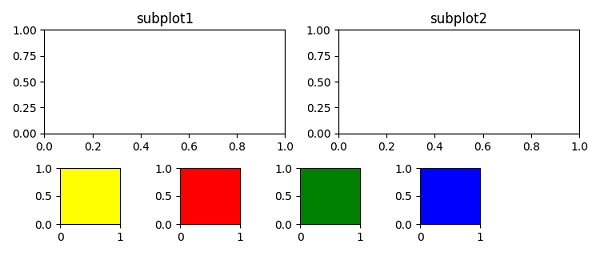## Defining

add_subplot − Add an axes to the figure as part of a subplot arrangement.

## Steps

• Create a new figure, or activate an existing figure, using the figure() method.

• Add an axes to the figure as part of a subplot arrangement where nrows=2, ncols=2. At index 1, add the title "subtitle1" and at index 2, add the title "subplot2".

• Create points for four rectangles and use add_axes() method to add an axes to the figure.

• To display the figure, use the show() method.

## Example

from matplotlib import pyplot as plt
plt.rcParams["figure.figsize"] = [7.50, 3.50]
plt.rcParams["figure.autolayout"] = True
fig = plt.figure()
plt.title("subplot1")
plt.title("subplot2")
rect1 = .1, .2, .1, .2
rect2 = .3, .2, .1, .2
rect3 = .5, .2, .1, .2
rect4 = .7, .2, .1, .2
plt.show()